Next: Examples Up: Modulation Previous: Waveshaping   Contents   Index

# Frequency and phase modulation

If a sinusoid is given a frequency which varies slowly in time we hear it as having a varying pitch. But if the pitch changes so quickly that our ears can't track the change--for instance, if the change itself occurs at or above the fundamental frequency of the sinusoid--we hear a timbral change. The timbres so generated are rich and widely varying. The discovery by John Chowning of this possibility [Cho73] revolutionized the field of computer music. Here we develop frequency modulation, usually called FM, as a special case of waveshaping [Leb79] [DJ85, pp.155-158]; the analysis given here is somewhat different [Puc01].

The FM technique, in its simplest form, is shown in Figure 5.8 (part a). A frequency-modulated sinusoid is one whose frequency varies sinusoidally, at some angular frequency, about a central frequency, so that the instantaneous frequencies vary betweenand, with parameterscontrolling the frequency of variation, andcontrolling the depth of variation. The parameters,, andare called the carrier frequency, the modulation frequency, and the index of modulation, respectively.

It is customary to use a simpler, essentially equivalent formulation in which the phase, instead of the frequency, of the carrier sinusoid is modulated sinusoidally. (This gives an equivalent result since the instantaneous frequency is the rate of change of phase, and since the rate of change of a sinusoid is just another sinusoid.) The phase modulation formulation is shown in part (b) of the figure.

We can analyze the result of phase modulation as follows, assuming that the modulating oscillator and the wavetable are both sinusoidal, and that the carrier and modulation frequencies don't themselves vary in time. The resulting signal can then be written asThe parameter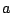, which takes the place of the earlier parameter, is likewise called the index of modulation; it too controls the extent of frequency variation relative to the carrier frequency. If, there is no frequency variation and the expression reduces to the unmodified, carrier sinusoid; asincreases the waveform becomes more complex.To analyse the resulting spectrum we can rewrite the signal as,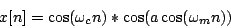We can consider the result as a sum of two waveshaping generators, each operating on a sinusoid of frequencyand with a waveshaping index, and each ring modulated with a sinusoid of frequency. The waveshaping functionis given by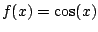for the first term and byfor the second.

Returning to Figure 5.4, we can predict what the spectrum will look like. The two harmonic spectra, of the waveshaping outputsand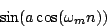have, respectively, harmonics tuned toand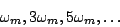and each is multiplied by a sinusoid at the carrier frequency. So there will be a spectrum centered at the carrier frequency, with sidebands at both even and odd multiples of the modulation frequency, contributed respectively by the sine and cosine waveshaping terms above. The index of modulation, as it changes, controls the relative strength of the various partials. The partials themselves are situated at the frequencieswhereAs with any situation where two periodic signals are multiplied, if there is some common supermultiple of the two periods, the resulting product will repeat at that longer period. So if the two periods are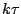and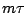, whereandare relatively prime, they both repeat after a time interval of. In other words, if the two have frequencies which are both multiples of some common frequency, so that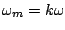and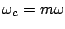, again withandrelatively prime, the result will repeat at a frequency of the common submultiple. On the other hand, if no common submultiplecan be found, or if the only submultiples are lower than any discernible pitch, then the result will be inharmonic.

Much more about FM can be found in textbooks [Moo90, p. 316] [DJ85, pp.115-139] [Bou00] and the research literature. Some of the possibilities are shown in the following examples.Next: Examples Up: Modulation Previous: Waveshaping   Contents   Index
Miller Puckette 2006-12-30